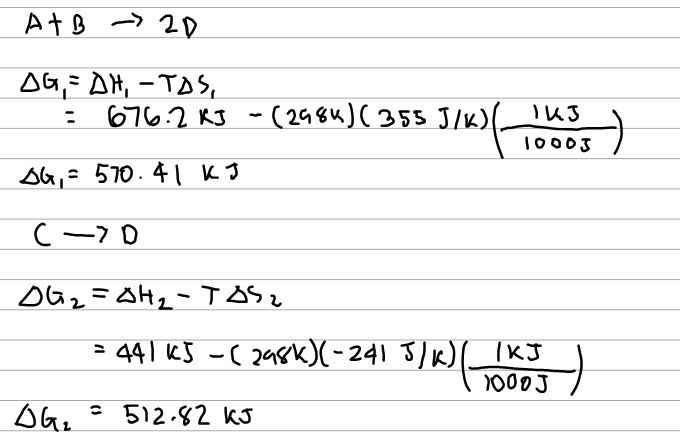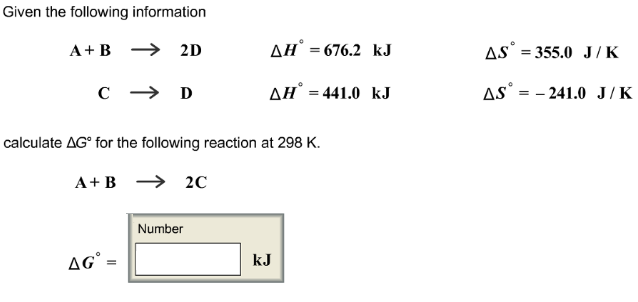# Problem: Given the following information A + B → 2D            ΔH° = 676.2 kJ                 ΔS° = 355.0 J/K C → D                    ΔH° = 44 1.0 kJ                 ΔS° = 241.0 J/K calculate ΔG° for the following reaction at 298 K. A + B → 2C

###### FREE Expert Solution87% (464 ratings)###### Problem Details

Given the following information

A + B → 2D            ΔH° = 676.2 kJ                 ΔS° = 355.0 J/K

C → D                    ΔH° = 44 1.0 kJ                 ΔS° = 241.0 J/K

calculate ΔG° for the following reaction at 298 K.

A + B → 2C